Pressure

(Redirected from Virial pressure)

Pressure ($p$) is the force per unit area applied on a surface, in a direction perpendicular to that surface, i.e. the scalar part of the stress tensor under equilibrium/hydrostatic conditions.

Thermodynamics

In thermodynamics the pressure is given by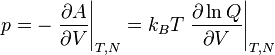$p = - \left.\frac{\partial A}{\partial V} \right\vert_{T,N} = k_BT \left.\frac{\partial \ln Q}{\partial V} \right\vert_{T,N}$

where$A$ is the Helmholtz energy function,$V$ is the volume,$k_B$ is the Boltzmann constant,$T$ is the temperature and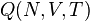$Q (N,V,T)$ is the canonical ensemble partition function.

Units

The SI units for pressure are Pascals (Pa), 1 Pa being 1 N/m2, or 1 J/m3. Other frequently encountered units are bars and millibars (mbar); 1 mbar = 100 Pa = 1 hPa, 1 hectopascal. 1 bar is 105 Pa by definition. This is very close to the standard atmosphere (atm), approximately equal to typical air pressure at earth mean sea level: atm, standard atmosphere = 101325 Pa = 101.325 kPa = 1013.25 hPa = 1.01325 bar

Stress

The stress is given by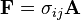${\mathbf F} = \sigma_{ij} {\mathbf A}$

where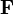${\mathbf F}$ is the force,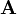${\mathbf A}$ is the area, and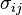$\sigma_{ij}$ is the stress tensor, given by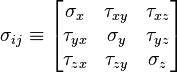$\sigma_{ij} \equiv \left[{\begin{matrix} \sigma _x & \tau _{xy} & \tau _{xz} \\ \tau _{yx} & \sigma _y & \tau _{yz} \\ \tau _{zx} & \tau _{zy} & \sigma _z \\ \end{matrix}}\right]$

where where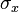$\ \sigma_{x}$,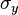$\ \sigma_{y}$, and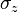$\ \sigma_{z}$ are normal stresses, and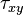$\ \tau_{xy}$,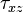$\ \tau_{xz}$,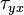$\ \tau_{yx}$,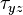$\ \tau_{yz}$,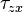$\ \tau_{zx}$, and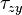$\ \tau_{zy}$ are shear stresess.

Virial pressure

The virial pressure is commonly used to obtain the pressure from a general simulation. It is particularly well suited to molecular dynamics, since forces are evaluated and readily available. For pair interactions, one has (Eq. 2 in ):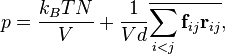$p = \frac{ k_B T N}{V} + \frac{ 1 }{ V d } \overline{ \sum_{i

where$p$ is the pressure,$T$ is the temperature,$V$ is the volume and$k_B$ is the Boltzmann constant. In this equation one can recognize an ideal gas contribution, and a second term due to the virial. The overline is an average, which would be a time average in molecular dynamics, or an ensemble average in Monte Carlo;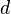$d$ is the dimension of the system (3 in the "real" world).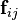${\mathbf f}_{ij}$ is the force on particle$i$ exerted by particle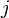$j$, and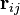${\mathbf r}_{ij}$ is the vector going from$i$ to$j$: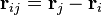${\mathbf r}_{ij} = {\mathbf r}_j - {\mathbf r}_i$.

This relationship is readily obtained by writing the partition function in "reduced coordinates", i.e.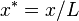$x^*=x/L$, etc, then considering a "blow-up" of the system by changing the value of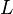$L$. This would apply to a simple cubic system, but the same ideas can also be applied to obtain expressions for the stress tensor and the surface tension, and are also used in constant-pressure Monte Carlo.

If the interaction is central, the force is given by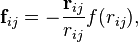${\mathbf f}_{ij} = - \frac{{\mathbf r}_{ij}}{ r_{ij}} f(r_{ij}) ,$

where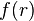$f(r)$ the force corresponding to the intermolecular potential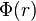$\Phi(r)$: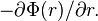$-\partial \Phi(r)/\partial r.$

For example, for the Lennard-Jones potential,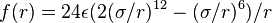$f(r)=24\epsilon(2(\sigma/r)^{12}- (\sigma/r)^6 )/r$. Hence, the expression reduces to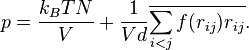$p = \frac{ k_B T N}{V} + \frac{ 1 }{ V d } \overline{ \sum_{i

Notice that most realistic potentials are attractive at long ranges; hence the first correction to the ideal pressure will be a negative contribution: the second virial coefficient. On the other hand, contributions from purely repulsive potentials, such as hard spheres, are always positive.

Pressure equation

For particles acting through two-body central forces alone one may use the thermodynamic relation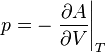$p = -\left. \frac{\partial A}{\partial V}\right\vert_T$

Using this relation, along with the Helmholtz energy function and the canonical partition function, one arrives at the so-called pressure equation (also known as the virial equation):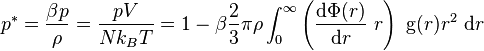$p^*=\frac{\beta p}{\rho}= \frac{pV}{Nk_BT} = 1 - \beta \frac{2}{3} \pi \rho \int_0^{\infty} \left( \frac{{\rm d}\Phi(r)} {{\rm d}r}~r \right)~{\rm g}(r)r^2~{\rm d}r$

where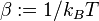$\beta := 1/k_BT$,$\Phi(r)$ is a central potential and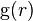${\rm g}(r)$ is the pair distribution function.